# NCERT Solutions Class 10 Science Chapter 12 Electricity

Ncert Solutions for class 10 subject Science Chapter 12 Electricityin pdf Best Free NCERT Solutions for class 1 to 12 in pdf NCERT Solutions, cbse board, Science, ncert Solutions for Class 10 Science, class 10 Science ncert solutions, Electricity, Class 10, ncert solutions chapter 12 Electricity, class 10 Science, class 10 Science ncert solutions, Science ncert solutions class 10, Ncert Solutions Class 10 Science Chapter 12 Electricity

## Exercise Chapter 12 Class 10 Science Electricity Ncert Solutions

Q 1: A piece of wire of resistance R is cut into five equal parts. These parts are then connected in parallel. If the equivalent resistance of this combination is R', then the ratio R/R' is –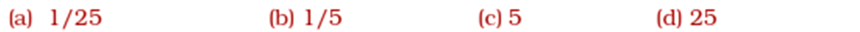Ans:
(d) Resistance of a piece of wire is proportional to its length. A piece of wire has a resistance R. The wire is cut into five equal parts.Therefore, resistance of each part =R/5
All the five parts are connected in parallel.
∴equivalent resistance (R') is given as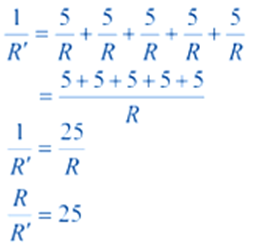Q 2: Which of the following terms does not represent electrical power in a circuit?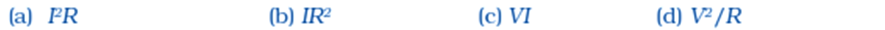Ans:
(b) Power cannot be represented by IR2.

Q 3: An electric bulb is rated 220 V and 100 W. When it is operated on 110 V, the power consumed will be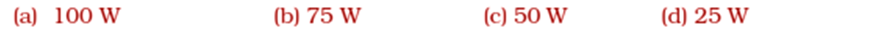Ans:
(d): 25w
Solution : R=V2/P < class="tab">= (220)2/100
= 484 Ω The resistance of the bulb remains constant
if the supply voltage is reduced to 110 V then Vʹ=110 V
the power consumed by it is given by the expression = Vʹ2/R
< class="tab">= (110)2/484 = 25w

Q 4: Two conducting wires of the same material and of equal lengths and equal diameters are first connected in series and then parallel in a circuit across the same potential difference. The ratio of heat produced in series and parallel combinations would be –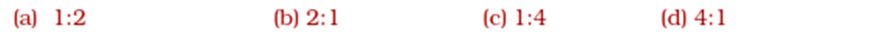Ans:
((c) Heat produced in the circuit is inversely proportional to the resistance R.
Let RS be the equivalent resistances of the wires if connected in series and RP be the equivalent resistances of the wires if connected in parallel
Then RS =R+R=2R
And1/ RP = 1/R +1/R
< class="tab">1/ RP = 2/R
OrRP =R/2
Now the ratio of heat produced is given by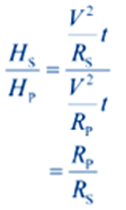< class="tab">= 1/4

Q 5: How is a voltmeter connected in the circuit to measure the potential difference between two points?
Ans:
A voltmeter must always be connected in parallel to measure the potential difference between two points.

Q 6: A copper wire has diameter 0.5 mm and resistivity of 1.6 × 10−8 Ω m. What will be the length of this wire to make its resistance 10 Ω? How much does the resistance change if the diameter is doubled?
Ans:
Area of cross-section of the wire, A =π (d/2) 2
Diameter= 0.5 mm = 0.0005 m
Resistance, R = 10 Ω
We know that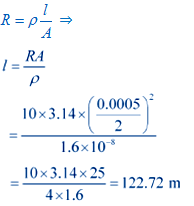∴ length of the wire = 122.72m
If the diameter of the wire is doubled, new diameter=2×0.5=1mm=0.001m
Let new resistance be Rʹ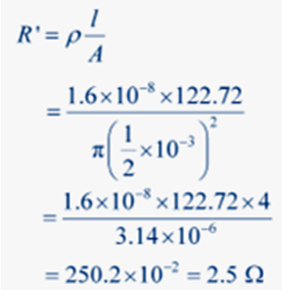∴ the new resistance is 2.5 Ω

Q 7: The values of current I flowing in a given resistor for the corresponding values of potential difference V across the resistor are given below −

 I (Amperes ) 0.5 1 2 3 4 V (volts) 1.6 3.4 6.7 10.2 13.2
Plot a graph between V and I and calculate the resistance of that resistor. Ans: The voltage is plotted on x-axis and current is plotted on y-axis. The values of the current for different values ofthe voltage are shown in the given table.

Ans:
The voltage is plotted on x-axis and current is plotted on y-axis. The values of the current for different values ofthe voltage are shown in the given table.

 I (Amperes ) 0.5 1 2 3 4 V (volts) 1.6 3.4 6.7 10.2 13.2

The IV characteristic of the given resistor is plotted in the following figure.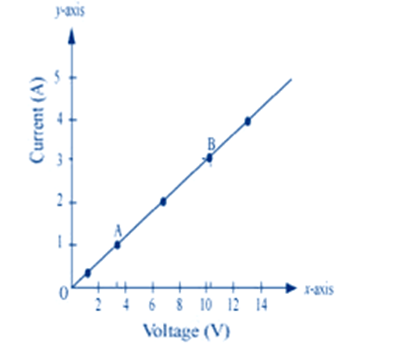Q 8: When a 12 V battery is connected across an unknown resistor, there is a current of 2.5 mA in the circuit. Find the value of the resistance of the resistor.
Ans:
We have potential difference (V) = 12V
Electric current (I)=2.5 mA= 2.4×10-3
According to Ohm’s Law (V) = IR Or R = V/I
$$R = {{12} \over {2.5 \times {{10}^{ - 3}}}} = 4.8 \times {10^3}\Omega$$Q 9: A battery of 9 V is connected in series with resistors of 0.2 Ω, 0.3 Ω, 0.4 Ω, 0.5 Ω and 12 Ω, respectively. How much current would flow through the 12 Ω resistor?
Ans:
There is no current ision occurring in a series circuit. Current flow through the component is the same.
Resistances are connected in series. Hence, the sum of the resistances will give the value of R. R = 0.2 + 0.3 + 0.4 + 0.5 + 12 = 13.4 Ω
Potential difference, V = 9 V
< class="fontmath">$I = {V \over R} = {{9V} \over {13.4\Omega }} = 0.671A$
∴ the current that would flow through the 12 Ω resistor will be 0.671 A.

Q 10: How many 176 Ω resistors (in parallel) are required to carry 5 A on a 220 V line?
Ans:
For x number of resistors of resistance 176 Ω,
Here Supply voltage, V = 220 V
Current, I = 5 A
Equivalent resistance of the combination = R, given as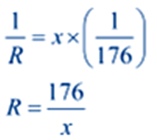From Ohm’s law,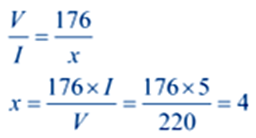∴four resistors of 176 Ω are required to draw the given amount of current.

Q 11: Show how you would connect three resistors, each of resistance 6 Ω, so that the combination has a resistance of (i) 9 Ω, (ii) 4 Ω.
Ans: (i) Two resistors in parallel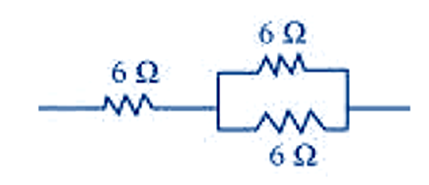Two 6 Ω resistors are connected in parallel. Their equivalent resistance will < class="fontmath"> \eqalign{& {1 \over {{R_p}}} = {1 \over 6} + {1 \over 6} \cr& {1 \over {{R_p}}} = {{1 + 1} \over 6} \cr& {1 \over {{R_p}}} = {2 \over 6} = {1 \over 3} \cr& \Rightarrow {R_p} = 3\Omega \cr}

The third 6 Ω resistor is in series with 3 Ω. Hence, the net resistance of the circuit is 6 Ω + 3 Ω = 9 Ω.
(ii) Two resistors in series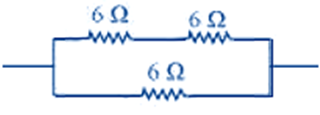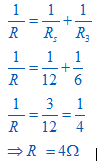∴ the total resistance is 4 Ω.

Q 12: Several electric bulbs designed to be used on a 220 V electric supply line, are rated 10 W. How many lamps can be connected in parallel with each other across the two wires of 220 V line if the maximum allowable current is 5 A?
Ans:
Resistance R1 of the bulb is given by the expression,
Supply voltage, V = 220 V
Maximum allowable current, I = 5 A
Rating of an electric bulb P=10watts
Because R=V2/P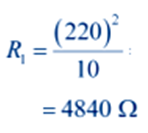According to Ohm’s law,
V = I R
Let R is the total resistance of the circuit for x number of electric bulbs
R=V/I
=220/5=44 Ω
Resistance of each electric bulb, R1=4840Ω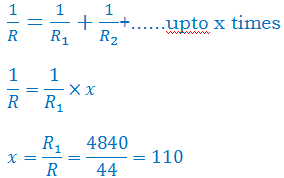∴ Number of electric bulbs connected in parallel are 110.

Q 13: A hot plate of an electric oven connected to a 220 V line has two resistance coils A and B, each of 24 Ω resistances, which may be used separately, in series, or in parallel. What are the currents in the three cases?
Ans:
Supply voltage, V = 220 V
Here Resistance of one coil, R =24 Ω
(i) Coils are used separately
According to Ohm’s law, V=I1R1
I1 is the current flowing through the coil
I1=V/R1=220/24=9.166A
∴ current flow through the coil when used separately is 9.16 A.
(ii) Coils are connected in series
R2=24 Ω+24 Ω=48 Ω
According to Ohm’s law
I2=V/R2=220/48=4.58A
the current flowing through the series circuit is 4.58A
(iii) Coils are connected in parallel
\eqalign{ & {1 \over {{R_3}}} = {1 \over {24}} + {1 \over {24}} \cr & {1 \over {{R_3}}} = {{1 + 1} \over {24}} \cr & {1 \over {{R_3}}} = {2 \over {24}} = {1 \over {12}} \cr & \Rightarrow {R_3} = 12\Omega \cr}

According to Ohm’s law
I3=V/R3=220/12=18.33A
the current flowing through the parallel circuit is 18.33A

Q 14: Compare the power used in the 2 Ω resistor in each of the following circuits: (i) a 6 V battery in series with 1 Ω and 2 Ω resistors, and (ii) a 4 V battery in parallel with 12 Ω and 2 Ω resistors.
(i) Potential difference (V) = 6 V
Here 1 Ω and 2 Ω resistors are connected in series.
∴ equivalent resistance of the circuit (R) = 1 + 2 = 3 Ω
According to Ohm’s law
V = IR
Or I=V/R=6/3=2A
the current through the circuit (I)=2A
Now the power used in the 2 Ω resistor
we know that Power = I2R
= 2×2×2=8W
(ii) Potential difference, V = 4 V
12 Ω and 2 Ω resistors are connected in parallel. The voltage across each component of a parallel circuit remains the same. Hence, the voltage across 2 Ω resistor will be 4 V. Power consumed by 2 Ω resistor is given by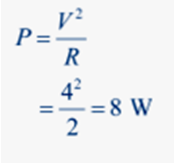∴ the power used by 2 Ω resistor is 8 W.

Q 15: Two lamps, one rated 100 W at 220 V, and the other 60 W at 220 V, are connected in parallel to electric mains supply. What current is drawn from the line if the supply voltage is 220 V?
Ans:
Both the bulbs are connected in parallel. Therefore, potential difference across each of them will be same that is 220 V, Current drawn by the bulb of rating 100 W is given by
I=P/V
=100/220=0.4545...A
Similarly, current drawn by the bulb of rating 60 W is given by
I=P/V
=60/220=0.2727...A
∴ the total current drawn from the line =0.4545+0.2727=0.727A

Q 16: Which uses more energy, a 250 W TV set in 1 hr, or a 1200 W toaster in 10 minutes?
Solution:

For T.V set
Power (P) = 250w, Time (t) = 1hr=60×60=3600s
For Toaster
Power (P) = 1200w, Time (t) = 10min=10×60=600s
We know that Energy (E) = P×t
∴ Energy consumed by a TV set = 250 × 3600 = 9 × 105J
∴ Energy consumed by a toaster = 1200 × 600 = 7.2× 105J
∴ the energy consumed by a 250 W TV set in 1 h is more than the energy
consumed by a toaster of power 1200 W in 10 minutes.

Q 17: An electric heater of resistance 8 Ω draws 15 A from the service mains 2 hours. Calculate the rate at which heat is developed in the heater.
Solution:

Here Given that Resistance of the electric heater (R) = 8 Ω
Current drawn (I)= 15 A
We know that P = I2R
= 15×15×8 $e = m{c^2}$
= 1800w or J/s
∴ Heat is produced by the heater at the rate of 1800 J/s.

Q 18: Explain the following.
(a) Why is the tungsten used almost exclusively for filament of electric lamps?
(b) Why are the conductors of electric heating devices, such as bread-toasters and electric irons, made of an alloy rather than a pure metal?
(c) Why is the series arrangement not used for domestic circuits?
(d) How does the resistance of a wire vary with its area of cross-section?
(e) Why are copper and aluminium wires usually employed for electricity transmission?

(a) Tungsten is an alloy which has very high melting point and very high resistivity so does not burn easily at a high temperature.
(b) The conductors of electric heating devices such as bread toasters and electric irons are made of alloy because resistivity of an alloy is more than that of metals which produces large amount of heat.
(c) In series circuits voltage is ided. Each component of a series circuit receives a small voltage so the amount of current decreases and the device becomes hot and does not work properly. Hence, series arrangement is not used in domestic circuits.
(d) Resistance (R) of a wire is inversely proportional to its area of cross-section (A), i.e. when area of cross section increases the resistance decreases or vice versa.
(e) Copper and aluminium are good conductors of electricity also they have low resistivity. So they are usually used for electricity transmission.

## In text Question Chapter 12 Class 10 Science Electricity Ncert Solutions

Ans: A continuous and closed path of an electric current is called an electric circuit. An electric circuit consists of electric devices, source of electricity and wires that are connected with the help of a switch.
<>Ans: We know that the a electron have charge of 1.6 × 10-19 C
∴ e =1.6 × 10-19 C
Total charge required 1Coulomb.
∴ q = 1C
Since q=ne
⇒ n =q/e

< class="fontmath">

= n = $\frac{1C}{1.6\times {{10}^{-19}}C}$

=6.25×1018
=6×1018 electrons.

Ans: Any source of electricity like battery, cell, power supply, etc. helps to maintain a potential difference across a conductor.
Ans: When 1 Joule of work is done to move a charge of amount 1 Coulomb from one place to another, then it is said that the potential difference between the two points is 1 Volt (V).
Ans: Factors on which the resistance of a conductor depends are:
(a) Length of the conductor
(b) Cross-sectional area of the conductor
(c) Nature of the material of the conductor
(d) Temperature

Ans: Current will flow more easily through a thick wire because the resistance is inversely proportional to the area of cross-section of the wire. Which means when the area of cross section of the wire increases then resistance decreases or vice versa. Ans: According to Ohm’s law
V = IR
⇒ I=V/R ---------- (1)
Now Potential difference is decreased to half
∴ New potential difference Vʹ=V/2
Resistance remains constant
So the new current Iʹ = Vʹ/R
= (V/2)/R
= (1/2) (V/R)
= (1/2) I = I/2

Ans: Coils of electric toasters and electric irons made of an alloy rather than a pure metal because the resistivity of an alloy is higher than the pure metal. Also at high temperatures, the alloys do not melt easily. Ans: Three cells of potential 2 V, each connected in series therefore the potential difference of the battery will be 2 V + 2 V + 2 V = 6V. The following circuit diagram shows three resistors of resistances 5 Ω, 8 Ω and 12 Ω respectively connected in series and a battery of potential 6 V and a plug key which is closed means the current is flowing in the circuit.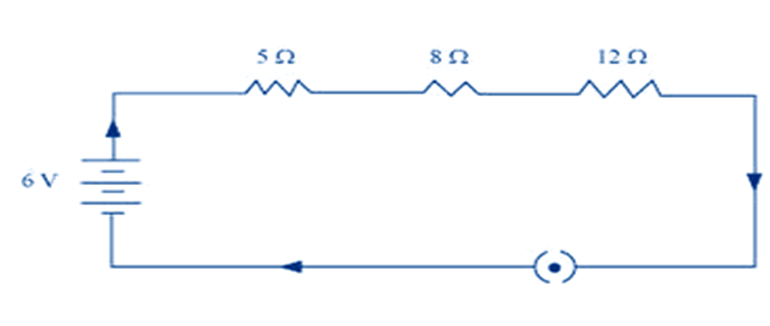Ans: An ammeter should be connected in the circuit in series with the resistors. To measure the potential difference across the resistor it should be connected in parallel, as shown in the following figure.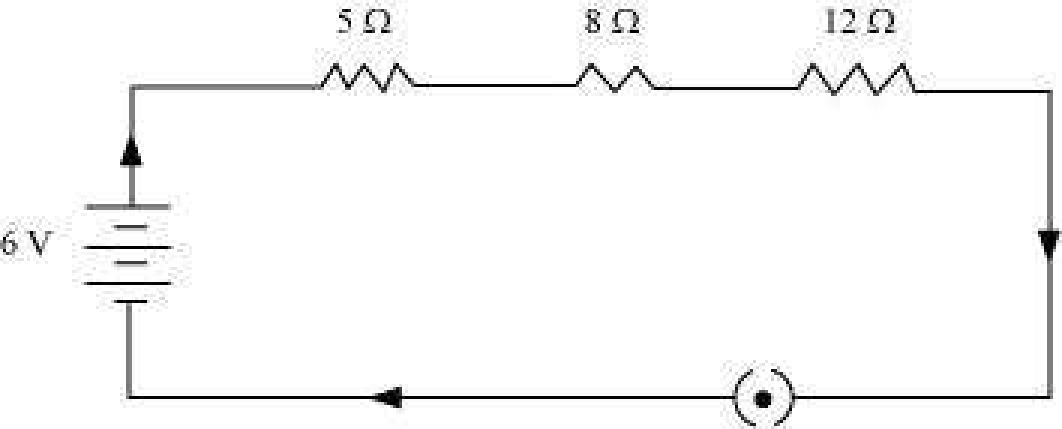According to Ohm’s law,
V = IR,
Now Potential difference (V) = 6 V
Let current flowing through the circuit = I
Resistance of the circuit, R = 5 + 8 + 12 = 25 Ω
Since I =V/R
= 6/25
= 0.24 A
Potential difference across 12 Ω resistor = V1
Current flowing through the 12 Ω resistor, I = 0.24 A
Therefore, using Ohm’s law, we obtain
V1 = IR
= 0.24×12=2.88 V
Therefore, the reading of the ammeter will be 0.24 A.
And the reading of the voltmeter will be 2.88 V. Ans: (a) Since 1 Ω and 106 Ω are connected in parallel then the equivalent resistance R will be
< class="fontmath">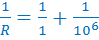⇒ R =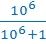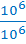=1 Ω
∴ Equivalent resistance is 1 Ω
(b) 1 Ω and 103Ω and 106Ω are in parallel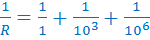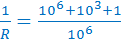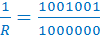⇒ R = 0.999 Ω
Therefore, equivalent resistance = 0.999 Ω Ans: Resistance of electric lamp (R1) = 100 Ω
Resistance of toaster (R2) = 5 0 Ω
Resistance of water filter (R3) = 500 Ω
Potential difference of the source, V = 220 V
Since all the resistance are in parallel then the equivalent resistance R of the circuit will be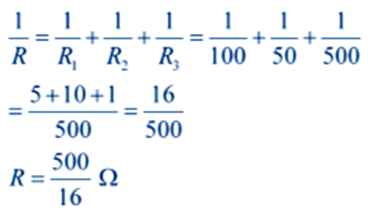According to Ohm’s law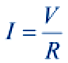Where current flowing through the circuit = I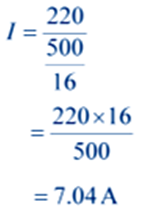All the three given appliances are drawing 7.04 A of current.
Therefore, current drawn by an electric iron connected to the same source of potential 220 V = 7.04 A
Let Rʹ be the resistance of the electric iron. According to Ohm’s law,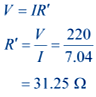Therefore, the resistance of the electric iron is 31.25 Ω and the current flowing through it is 7.04 A. Ans: When the electric appliances are connected in parallel then each device get same potential difference also the total effective resistance is reduced.

Ans: There are three resistors of resistances 2 Ω, 3 Ω, and 6 Ω respectively.
(a) If we connect the given three resistors in the following way then we get net resistance as 4Ω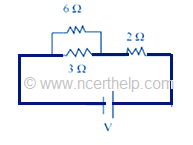Here, 6 Ω and 3 Ω resistors are connected in parallel. Therefore, their equivalent resistance will be given by

< class="fontmath">\begin{align}& ~\frac{1}{R}=\frac{1}{{{R}_{1}}}+\frac{1}{{{R}_{2}}} \\& \frac{1}{R}=\frac{1}{6}+\frac{1}{3} \\& \frac{1}{R}=\frac{1+2}{6} \\& \frac{1}{R}=\frac{3}{6}=\frac{1}{2} \\ \end{align} ⇒R=2 Ω
This equivalent resistor of resistance 2Ω is connected to a 2Ω resistor in series.
Therefore, equivalent resistance of the circuit = 2Ω + 2Ω =4Ω
Hence, the total resistance of the circuit is 4Ω.(b) If we connect the given three resistors in the following way then we get net resistance as 1Ω. Here all the resistors are connected in parallel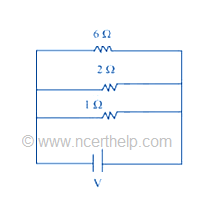∴ their equivalent resistance will be given as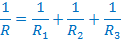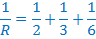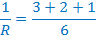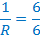R=1Ω
Therefore, the net equivalent resistance of the circuit is 1Ω. Ans: The heating element of the heater is made up of alloy which has very high resistance so when current flows through the heating element, it becomes too hot and glows red. But the resistance of cord which is usually of copper or aluminium is very law so it does not glow. Ans: Given Charge (Q) =96000C
Time (t)=1hr=60×60=3600s
Potential difference V=50volts
Now we know that H= VIt
So we have to calculate I first
As I=Q/t
∴ I=96000/3600=80/3 A
Now we can calculate the heat generated
H=J
=50×80×1200J
=4800000J
=4.8×106J.

Ans: The rate at which energy is delivered by a current is the power of the appliance.

Ans: Given that electric current (I) = 5A, Potential difference (V) =220V
Time (t) =2h=2×60×60s=7200s
Now Power (P) is given by the expression
< class="tab">P=VI
=220×5watts
=1100w Now Energy consumed by the motor
< class="tab">H= Pt
=1100×7200J
=7.92 × 106J

Ans: The unit of current is ampere (A). 1 Ampere is defined as the flow of 1 Coulomb of charge through a wire in 1 second.
Ans: Factors on which the resistance of a conductor depends are:
(a) Length of the conductor
(b) Cross-sectional area of the conductor
(c) Nature of the material of the conductor
(d) Temperature

Ans: Given resistances R1 =4 Ω, R2=8 Ω, R3=12Ω, and R4=24Ω.
(a) If these coils are connected in series, then the equivalent resistance will
be the highest, as R=R1+R2+R3+R4
∴ R=4+8+12+24 = 24Ω
(b) If these coils are connected in parallel, then the equivalent resistance will be the lowest, given by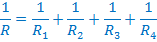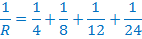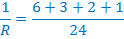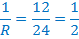⇒R=2 Ω
∴ 2 Ω is the lowest total resistance.

Ans: (a) In the said table we can see that the resistivity of mercury is more than that of iron. Therefore iron is a better conductor than mercury.
(b) In the said table we can see that the resistivity of silver is the lowest among the all materials. Hence, it is the best conductor. Ans: Given that Resistance(R) = 20 Ω, Electric current (I)= 5 A and time(t)= 30 s
Now we know that H= I2Rt
= 5×5×20×30
= 15000J=1.5×104J
∴ The heat developed in 30s is 1.5×104J

Ans: The energy given to each coulomb of charge is equal to the amount of work which is done in moving it. Now we know that Potential difference =Work done / Charge ∴ Work done = Potential difference × charge Where, Charge = 1 C and Potential difference = 6 V ∴ Work done = 6×1 = 6 Joule.

### NCERT Books Free Pdf Download for Class 5, 6, 7, 8, 9, 10 , 11, 12 Hindi and English Medium

 Mathematics Biology Psychology Chemistry English Economics Sociology Hindi Business Studies Geography Science Political Science Statistics Physics Accountancy